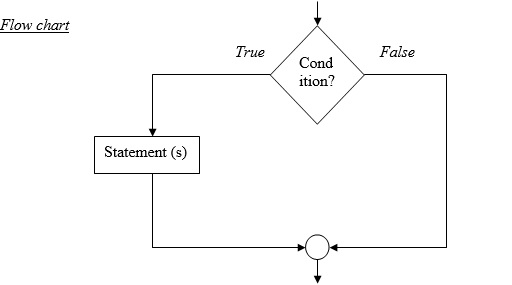# Explain ‘simple if’ statement in C language

CServer Side ProgrammingProgramming

‘if’ keyword is used to execute a set of statements when the logical condition is true.

## Syntax

The syntax is given below −

if (condition){
Statement (s)
}## Working of ‘simple if’ statement

• The statement inside the if block are executed only when condition is true, otherwise not.

• If we want to execute only one statement when condition is true, then braces ({}) can be removed. In general, we should not omit the braces even if, there is a single statement to execute.

• When the condition is true the braces ({}) are required to execute more than one statement.

## Example

Given below is the C program to execute If conditional operators −

Live Demo

#include<stdio.h>
void main (){
int a=4;
printf("Enter the value of a: ");
scanf("%d",&a);
if(a%2==1){
printf("a is odd number");
}
Return 0;
}

## Output

You will see the following output −

Run 1: Enter the value of a: 56
a is even number
Run2: Enter the value of a: 33

Here, if condition becomes false, as a result, statement inside the if block is skipped.